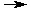Estimation of pH - Background Information

## Acids and Bases

A simple definition for an acid is "any substance that, when dissolved in water, produces hydronium ions (H3O+)". Hydronium ions are produced when an H+ ion from a proton donor reacts with water. For example, when hydrochloric acid is dissolved in water the following reaction occurs:

HCl(aq) + H2O(l)H3O+(aq) + Cl-(aq)

Bases can be defined as "any substance that, when dissolved in water, generates hydroxide ions (OH-)". Hydroxide ions are produced under two conditions. First, they are produced when an ionic compound containing hydroxide such as sodium hydroxide dissolves in water.

NaOH(s)Na+(aq) + OH-(aq)

Second, hydroxide ions are produced when a substance like ammonia accepts a proton from water.

NH3(aq) + H2O(l)NH4+(aq) + OH-(aq)

In all aqueous (water) solutions, the product of the hydronium ion and hydroxide ion concentrations is a constant. If the concentrations are expressed in molarity (indicated by square brackets) the constant is called Kw and is equal to 1 x 10-14 at 25oC. The formula appears below.

[H3O+] [OH-] = Kw = 1 x 10-14

In pure water and in neutral solutions, the [H3O+] and [OH-] are equal. Therefore, [H3O+] = [OH-] = 1 x10-7.

However, this can be altered by the addition of an acid or base. If an acid is added to pure water the concentration of hydronium ions in the solution increases. In order for [H3O+] [OH-] to remain constant the hydroxide ion concentration must decrease. As a result [H3O+] > [OH-] and the solution is said to be acidic. If a base is added to pure water, the reverse is true. When the concentration of hydroxide ions in a solution increases the hydronium ion concentration must decrease. In this case [H3O+] < [OH-] and the solution is considered basic.